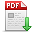# 张卫东,赵凤霞,苏清磊,等.激光扫平仪垂直扫平误差测量方法研究[J].郑州大学学报(工学版),2023,44(01):77-82. 　Measuring Vertical Error of the Rotating Laser[J].Journal of Zhengzhou University (Engineering Science),2023,44(01):77-82. 点击复制 激光扫平仪垂直扫平误差测量方法研究() 分享到： var jiathis_config = { data_track_clickback: true };

44卷

2023年01期

77-82

2022-12-06

## 文章信息/Info

Title:
Measuring Vertical Error of the Rotating Laser

A

Abstract:
In order to test the vertical accuracy of the vertical level plane about the rotating laser, the vertical level error of the laser level was analyzed. Using the sine and cosine theorems of spherical triangles, the calculation formula of total deviation of the rotating axis from the axis of the rotating laser was deduced. The mathematical model of the deflective deviation with the total deviation of the rotating axis from the axis was established. A special test device with double light pipes based on horizontal axis symmetry was built, and a scheme was designed to first measure the error component separately and then synthesize the vertical error, and experiments were carried out to verify it. The results showed that the maximum difference was no more than 6. 3" obtained in our scheme in different instrument poses, which was better than the maximum difference of 16. 5" in the traditional scheme, which proved that the designed scheme was technically feasible, and the mathematical model was accurate and reliable.